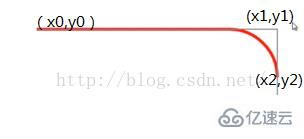# H5+Canvas的使用示例

`<canvas id='canvas'></canvas>`

```var convas=document.getElementById('canvas');
var context=canvas.getContext('2d');```

canvas是基于状态的绘图环境

```context.moveTo(x,y); 挪到笔头
context.lineTo(x,y);按着画笔移动 （可以结合使用moveTo画不相连的线段）moveTo lineTo +moveTo lineTo
context.lineWith=10;    canvas是基于状态的，它不会创建一个直线对象。我们是对canvas上下文进行其他设置。读完所以设置后再绘图
context.strokeStyle='red';   这里还有其他的样式，不仅仅只有颜色。
context.stroke();
context.beginPath();  开始一个全新的canvas状态。之前对context的设置不会因为beginPath()而改变。新的设置会覆盖之前的设置
context.closePath();  使用线段绘制封闭的图形。可以去掉最后一个lineTo。closePath()会完成封闭这个动作。
context.fillStyle='green';
context.fill();  建议在进行完所有的设置之后再fill和stroke。不然fill会覆盖一部分的线段宽度，会由10变成5;```

```context.rect(x,y,width,height);绘制矩形的路径
context.fillRect(x,y,width,height);使用当前fillStyle绘制矩形 ( 颜色值可以使用css认同的所有颜色值包括rgb、rgba、hsl、hsla)
context.strokeRect(x,y,width,height);使用当前的strokeStyle绘制矩形```

```lineWidth=10;
lineCap=''; 线段的2段的样式。butt(default)、round圆头、square方头。round和squqre比默认的长线条宽度的一半（5）的的长度。
lineJoin;线段与线段之间连接的样式。miter(default)、bevel(斜截)、round(圆头)```

```context.translate(x,y);移动坐标系
context.rotate(deg);旋转坐标系弧度制
context.scale(x,y);缩放坐标系--->需要注意的是，它也会对坐标数值，边框宽度属性等进行缩放，使用时需要谨慎选择。```

```context.save();
context.translate(x,y);
context.restore();```

```contex.transform(a,b,c,d,e,f);   a、d-水平垂直缩放；b、c水平垂直倾斜；e、f水平垂直位移。
context.transform(1,0,0,1,0,0);基本矩阵，图形不发生任何变化。

context.setTransform(a,b,e,d,e,f)。覆盖之前的transform设置，使用当前的transform设置，这样我们就很清楚当前具体transform变换。
context.fillStyle   ---填充样式  之  渐变
linearGradient:
var grd=context.creatLinearGradient(xStart,yStrart,xEnd,yEnd);   定义渐变色。在（xEnd,yEnd）点之后，填充的是最后的颜色值。
grd.addColorStop(stop,color);(stop的值，0-1的浮点数)   一般多个颜色断点配合使用，从而实现多颜色的渐变
context.fillStyle=grd;
context.fill();
radialGradient;
var grd=context.createRadialGradient(x0,y0,r0,x1,y1,r1); 定义2个同心圆，（x0,y0）位置的半径r0
gra.addColorStop(stop,color);
context.fillStyle=grd;
context.fill();
createPattern
var pattern=context.createPattern(img || canvas || video , repeat-style);
Img的值可以使一张图片，也可以使一个canvas画布，甚至是一段video
repeat-style的值：no-repeat、repeat-x、repeat-y、repeat
context.fillStyle=pattern;
context.fill();```

`context.arc(centerX , centerY , radius , startAngle , endAngle , anticlockwise=false)，`

contex.arcTo(x1 , y1 ,  x2 , y2 , radius);```ctx.moveTo(x0,y0);
ctx.quadraticCurveTo(x1，y1，x2，y2);```

```ctx.moveTo(x0,y0);
ctx.bezierCurveTo(x1，y1，x2，y2，x3，y3)```

canvas中文字的绘制

```ctx.font = "bold 40px Arial";
ctx.fillStyle=;
ctx.fillText(string ，x ，y，[maxlen]);在（x,y）位置绘制string 这个字符串
ctx.strokeStyle=;
ctx.strokeText(string，x，y，[maxlen]);在（x，y）位置绘制string 这个字符串，这个文字只有文字的边框，并没有填充```

string 这个字符串如果设置了maxlen这个可选参数，那么这段文字就会强制压缩在maxlen这个宽度中。

```ctx.font="20px sans-serif"(默认)，如果需要设置，只是这2个值
font：font-style、font-varient、font-weight、font-size、font-family（一个5个属性，和css基本相似）
font-style:normal、italic(斜体)、oblique（倾斜字体）。一般的web页面中italic和oblique是一样的，除非使用自己导入的倾斜字体。
font-varient：normal、small-caps(使用小型大写字母 替代 小写字母，大小和小写一样，只是他是大写的)
font-weight：lighter、normal、bold、bolder。一般浏览器，前2者一样，后2者一样。
font-size：px、em、%、large等
font-family：支持设置多种字体备用、支持@font-face、支持web安全字体
ctx.textAlign=left、center、right。```

ctx.textBaseLine=top、middle、bottom;

ctx.measureText(string).width-------根据对font设置之后，返回一个一段文字渲染的宽度

```ctx.shadowColor     --->CSS接受的颜色值均可
ctx.shadowOffsetX   --->x、y方向上的偏移    可为负值
ctx.shadowOffsetY
ctx.shadowBlur   --->模糊  0--> 越大越模糊```

canvas绘制 均可设置shadow阴影

global属性  对全局设置

```ctx.globalAlpha=1(default)   对全局设置透明度
ctx.globalCompositeOperation=   前后绘制图形重叠部分的样式     共有11种样式
"source-over"(default)  : 后绘制的图形覆盖前绘制的图形（默认）
"destination-over":前绘制的图形覆盖后绘制的图形```

11种样式如下:

```source-oversource-atopsource-insource-out
destination-over destination-atopdestination-indestination-out```

lighter(颜色计算)copy（只保留后者）xor(清除重叠部分)

ctx.beginPath();

ctx.arc(400,400,200,0,Math.PI*2);

ctx.clip();    --->有绘制了一个圆形的剪辑区域

```<!DOCTYPE html>
<html lang="en">
<head>
<meta charset="UTF-8">
<title>canvas之globalCompositeOperation</title>
<style type="text/css">
body{
background-color: #ccc;
}
</style>
</head>
<body>
<canvas id="canvas" style="display: block;margin: 50px auto;border:1px solid #ccc; background-color: #FFF"></canvas>
</body>
<script type="text/javascript">
var canvas=document.getElementById('canvas');
var WIDTH=1500;
var HEIGHT=800;
canvas.width=WIDTH;
canvas.height=HEIGHT;
var CIRCLES=[];
var COLORS=["0","1","2","3","4","5","6","7","8","9","a","b","c","d","e","f"];
var NUMS=220;
var SPEEDX=16;
var SPEEDY=8;
var RADIUS=25;
window.onload=function(){
if(canvas){
var context=canvas.getContext('2d');
gloablCompositeOperation(context);
}else{
console.log('浏览器不支持canvas，请更新浏览器！')
}
}
function gloablCompositeOperation(context){
context.clearRect(0, 0, WIDTH, HEIGHT);
context.globalCompositeOperation="xor";
context.globalAlpha=1;
addCircles(CIRCLES,NUMS);
paintCircles(context,CIRCLES);
//setInterval(run(context),90);	  //setInterval、setTimeout不能直接传入带参数的方法调用。 一般有2中方法，1、使用匿名方法包装下。
var interval=setInterval(function(){
go(context);
},90);
}
/*function run(context){	//2、定义一个带参数,返回一个无参方法，在返回的无参方法中调用之前带参方法。
return function(){
go(context);
}
}*/
function go(context){
updateCirCles(CIRCLES);
paintCircles(context,CIRCLES);
}
/*绘制一个圆对象*/
function paintCircles(ctx ,CIRCLES){
ctx.clearRect(0, 0, WIDTH, HEIGHT);
for(var i=0 ; i<CIRCLES.length ; i++){
ctx.beginPath();
ctx.arc(CIRCLES[i].x,CIRCLES[i].y,CIRCLES[i].r,0,Math.PI*2);
ctx.closePath();
ctx.fillStyle=CIRCLES[i].color;
ctx.fill();
}
}
/*随机生成新的圆对象，加入到数组*/
function addCircles(CIRCLES,num){
for(var i=0 ; i<num ;i++){
var circle={};
var radius=(1+Math.random())*RADIUS;
var	cX=Math.random()*WIDTH;
var	cY=Math.random()*HEIGHT;
cX=cX<radius?radius:cX;
cY=cY<radius?radius:cY;
cX=cX>(WIDTH-radius)?(WIDTH-radius):cX;
cY=cY>(HEIGHT-radius)?(HEIGHT-radius):cY;
circle.x=cX;
circle.y=cY;
circle.r=radius;
circle.color=createRandomRGBColor();
circle.vX=(Math.floor(Math.random()*10)%2)==0?SPEEDX:(-1)*SPEEDX;
circle.vY=(Math.floor(Math.random()*10)%2)==0?SPEEDY:(-1)*SPEEDY;
circle.vX=circle.vX+Math.random()*6;
circle.vY=circle.vY+Math.random()*3;
CIRCLES[i]=circle;
}
}
function updateCirCles(CIRCLES){
for(var i=0 ; i<CIRCLES.length ; i++){
var width=WIDTH-CIRCLES[i].r;
var height=HEIGHT-CIRCLES[i].r;

if(CIRCLES[i].x + CIRCLES[i].vX>width){
CIRCLES[i].x=CIRCLES[i].x;
CIRCLES[i].vX=CIRCLES[i].vX*(-1);
}else	if(CIRCLES[i].y + CIRCLES[i].vY>height){
CIRCLES[i].y=CIRCLES[i].y;
CIRCLES[i].vY=CIRCLES[i].vY*(-1);
}else	if(CIRCLES[i].x + CIRCLES[i].vX<CIRCLES[i].r){
CIRCLES[i].x=CIRCLES[i].x;
CIRCLES[i].vX=CIRCLES[i].vX*(-1);
}else	if(CIRCLES[i].y + CIRCLES[i].vY<CIRCLES[i].r){
CIRCLES[i].y=CIRCLES[i].y;
CIRCLES[i].vY=CIRCLES[i].vY*(-1);
}else{
CIRCLES[i].x=CIRCLES[i].x + CIRCLES[i].vX;
CIRCLES[i].y=CIRCLES[i].y + CIRCLES[i].vY;
}
}
}
//随机生成rgb颜色值"#123abc"
function createRandomRGBColor(){
var color="";
for(var i=0 ; i<6 ; i++){
color+=COLORS[Math.floor(Math.random()*COLORS.length)];
}
return "#"+color;
}
//随机生成rgba颜色值"#123abc"
function createRandomRGBAColor(){
var color="";
for(var i=0 ; i<3 ; i++){
color+=Math.floor(Math.random()*255)+",";
}
return "rgba("+color+Math.random()+")";  //"rgba("+color+Math.random()+")"
}
</script>
</html>```